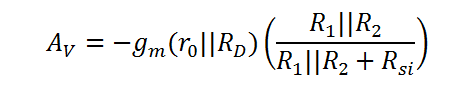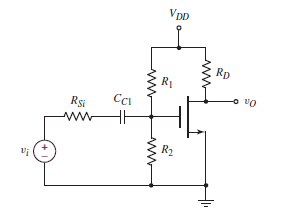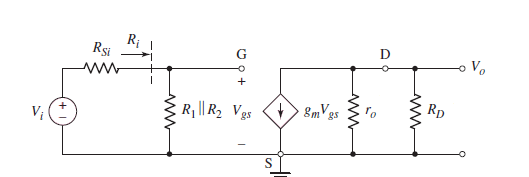# Small Signal Analysis of Common Source (CS) MOSFET Amplifier

This CalcTown calculator calculates the small signal voltage gain of a MOSFET amplifier.

* Please note that body effect has been taken into consideration only for calculating the small signal output resistance and not for the current calculation in DC analysis.

K-Ohm
K-Ohm
K-Ohm
K-Ohm
uA/V2
V-1

#### Result

K-Ohm
uA/V
V/V